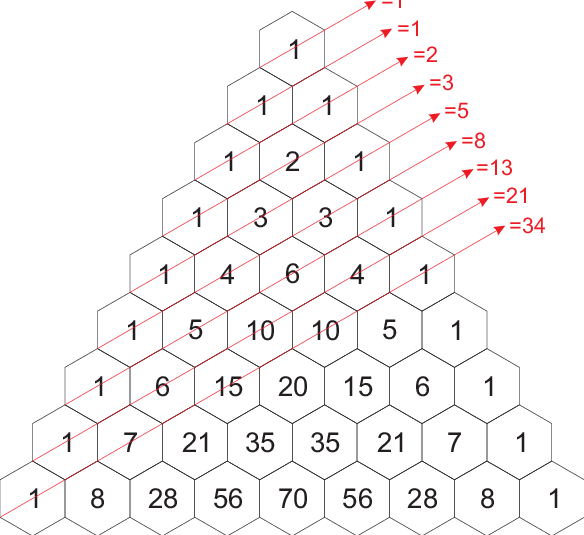In this blog we will learn how to create a fibonacci sequence using python.But before that,

What is Fibonacci Sequence
Steps Involved in writing fibonacci sequence program
Fibonacci Program In Python Using While Loop
Fibonacci Program In Python Using Recursions
Fibonacci Program In Python With Dynamic Programming

Fibonacci sequence follows a pattern where each number is the sum of the sum of the two preceding ones, starting from 0 and 1. That is,### Fibonacci sequence

Here are the first few fibonacci numbers of the sequence…

0, 1, 1, 2, 3, 5.……

The nth term in the sequence is the sum of (n-1)th and (n-2)th term.

Checkout this Online Python Course by FITA. FITA provides a complete Python course where you will be building real-time projects like Bitly and Twitter bundled with Career support.

Now let’s write programs using various methods to print the fibonacci terms until the input number.

Fibonacci Program In Python Using Recursions

 def fib(num):     if num <= 1:          return num     else:          return (fib(num – 1) + fib(num – 2)) nu= int(input(“Enter the number of terms you want: “)) if nu <= 0:    print(“Give a natural number”) else:    for n in range(nu):         print(fib(n),end=’,’)

Output

 Enter the number of terms you want: 4 0,1,1,2,

Steps involved in the above program:

Define a function to return fibonacci numbers.
Since the first term of the fibonacci sequence is 1, return 0 if the input is 1.
Return 1 if the input is 2, since the second term in sequence is 1.
Else call the function recursively for the value (n – 2) + (n – 1).

Fibonacci Program In Python With Dynamic Programming

 FibTerms = [0, 1] def fib(n):      if n <= len(FibTerms):             return FibTerms[n – 1]      else:             term = fib(n – 1) + fib(n – 2)             FibTerms.append(term)      return term n=int(input(‘How many terms do you want’)) for i in range(n):       print(fib(i),end=’,’)

Output

 Enter the number of terms you want: 9 1,0,1,1,2,3,5,8,13,

Steps involved in the above code:

Initialise a list to store the fibonacci number in the sequence.
Define a function to return the fibonacci number.
Check if the list has the number of terms as of input using len.
If the len of the list is less than or equal to the input, return the available numbers from the list.
Else, calculate the fibonacci number using recursion and add to the list.

Fibonacci Program In Python Using While Loop

 number = int(input(“How many terms? “)) n1, n2 = 0, 1 count = 0 if number <= 0:       print(“Enter a positive integer”) elif number == 1:       print(n1) else:       while count < number:              print(n1,end=’,’)              sum= n1 + n2              n1,n2 = n2,sum              count += 1

Steps involved in the above program

Take the input.
Initialise the first(n1) and second(n2) term with 0 and 1 and a count with 0.
If the number of terms are more than 2, use a while loop to find the get subsequent terms with a condition that input is bigger than count.